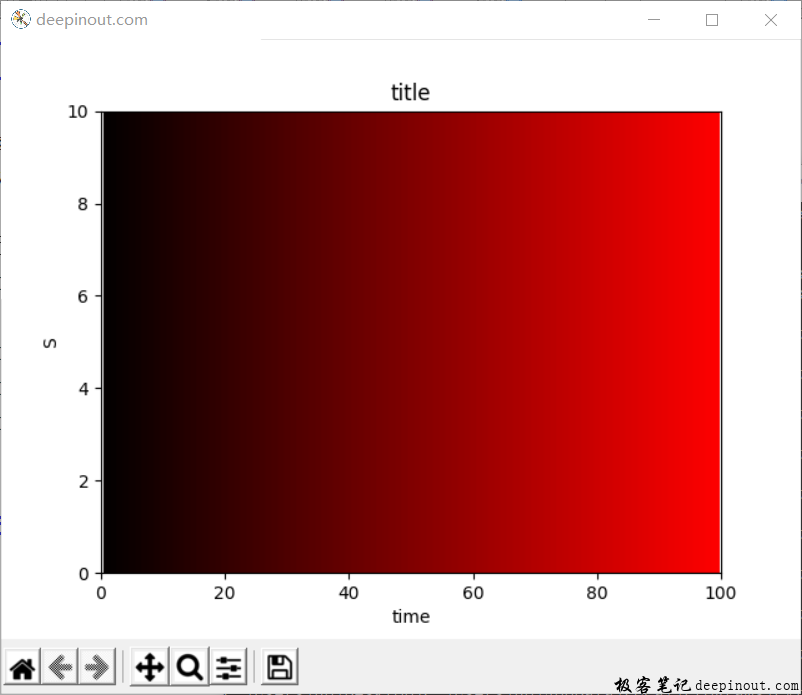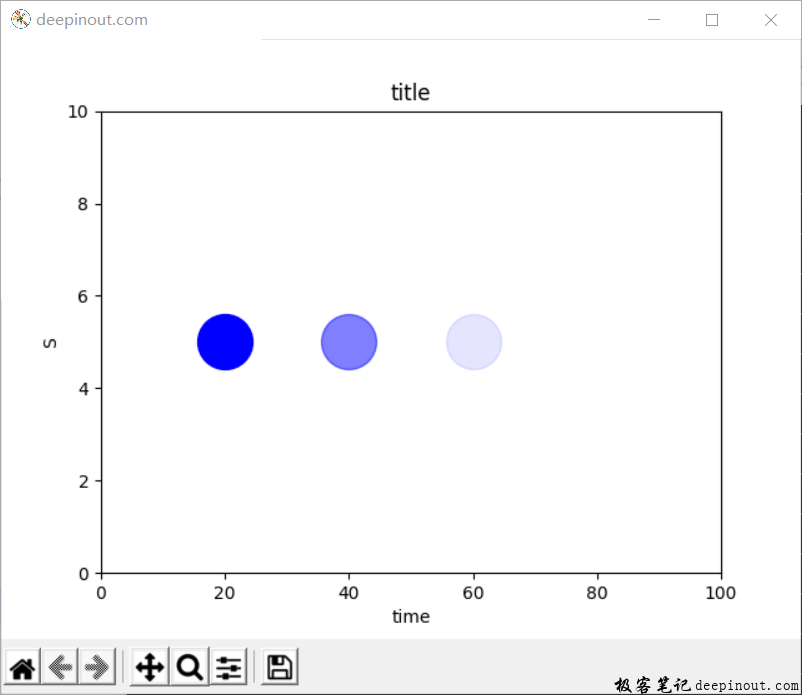# Matplotlib 颜色

• ‘k’表示黑色；
• ‘b’表示蓝色
• ‘c’表示青色
• ‘g’表示绿色
• ‘m’表示洋红
• ‘r’表示红色
• ‘y’黄色
• ‘gray’或’grey’代表灰色
• ‘lightgray’或’lightgrey’代表浅灰``plt.plot([x1, x2], [y1, y2], linewidth = lw, color = ‘color’, linestyle = ‘ls’)``

``````import matplotlib.pyplot as plt
import numpy as np

x1, x2 = 0, 100
y1, y2 = 0, 10

fig = plt.figure('deepinout.com')
plt.axis([x1, x2, y1, y2]) #

plt.xlabel('time')
plt.ylabel('S')
plt.title('title')

for x in np.arange(1, 100, 1):
r = x/100
g = 0
b = 0
plt.plot([x, x], [0, 10], linewidth = 5, color = (r, g, b))

plt.show()````````````import matplotlib.pyplot as plt
import numpy as np

x1, x2 = 0, 100
y1, y2 = 0, 10

fig = plt.figure('deepinout.com')

plt.axis([x1, x2, y1, y2]) #

plt.xlabel('time')
plt.ylabel('S')
plt.title('title')

plt.scatter(20, 5, s = 1000, color = 'b', alpha = 1)
plt.scatter(40, 5, s = 1000, color = 'b', alpha = 0.5)
plt.scatter(60, 5, s = 1000, color = 'b', alpha = 0.1)

plt.show()``````

• 微信订阅
• 回顶